Balbharti Maharashtra State Board 11th Maths Book Solutions Pdf Chapter 3 Permutations and Combination Miscellaneous Exercise 3 Questions and Answers.

## Maharashtra State Board 11th Maths Solutions Chapter 3 Permutations and Combination Miscellaneous Exercise 3

(I) Select the correct answer from the given alternatives.

Question 1.
A college offers 5 courses in the morning and 3 in the evening. The number of ways a student can select exactly one course, either in the morning or in the evening is
(A) 5
(B) 3
(C) 8
(D) 15
(C) 8
Hint:
Number of ways to select one course from available 8 courses
(i.e., 5 courses in the morning and 3 in the evening) = 5 + 3 = 8

Question 2.
A college has 7 courses in the morning and 3 in the evening. The possible number of choices with the student if he wants to study one course in the morning and one in the evening is
(A) 21
(B) 4
(C) 42
(D) 10
(A) 21
Hint:
Number of ways to select one morning and one evening course = 7C1 × 3C1 = 21Question 3.
In how many ways can 8 Indians and, 4 American and 4 Englishmen can be seated in a row so that all persons of the same nationality sit together?
(A) 3! 8!
(B) 3! 4! 8! 4!
(C) 4! 4!
(D) 8! 4! 4!
(B) 3! 4! 8! 4!
Hint:
8 Indians take their seats in 8! ways, 4 Americans take their seats in 4! ways, 4 Englishmen take their seats in 4! ways.
Three groups of Indians, Americans and Englishmen can be permuted in 3! ways.
Required number = 3! × 8! × 4! × 4!

Question 4.
In how many ways can 10 examination papers be arranged so that the best and the worst papers never come together?
(A) 9 × 8!
(B) 8 × 8!
(C) 9 × 9!
(D) 8 × 9!
(D) 8 × 9!
Hint:
Arrange 8 papers in 8! ways and two papers in 9 gaps are arranged in 9P2 ways.
Required number = 8! 9P2
= 8! × 9 × 8
= 9! × 8

Question 5.
In how many ways 4 boys and 3 girls can be seated in a row so that they are alternate.
(A) 12
(B) 288
(C) 144
(D) 256
(C) 144
Hint:
B G B G B G B
4 boys take their seats in 4! ways.
3 girls take their seats in 3! ways.
Required number = 4! × 3!
= 24 × 6
= 144Question 6.
Find the number of triangles which can be formed by joining the angular points of a polygon of 8 sides as vertices.
(A) 16
(B) 56
(C) 24
(D) 8
(B) 56
Hint:
A triangle is obtained by joining three vertices.
Number of ways of selecting 3 vertices out of 8 vertices = 8C3
= $$\frac{8 \times 7 \times 6}{1 \times 2 \times 3}$$
= 56

Question 7.
A question paper has two parts, A and B, each containing 10 questions. If a student has to choose 8 from part A and 5 from part B, in how many ways can he choose the questions?
(A) 320
(B) 750
(C) 40
(D) 11340
(D) 11340
Hint:
Number of ways to choose 8 questions from Part A and 5 from Part B = 10C8 × 10C5
= 10C2 × 10C5
= 45 × 252
= 11340

Question 8.
There are 10 persons among whom two are brothers. The total number of ways in which these persons can be seated around a round table so that exactly one person sits between the brothers is equal to:
(A) 2! × 7!
(B) 2! × 8!
(C) 3! × 7!
(D) 3! × 8!
(B) 2! × 8!
Hint:
Select a person from 8 people (i.e., the people excluding two brothers).
This is done in 8 ways.
2 brothers sit adjacent to the selected person on two sides, they may interchange their seats.
Remaining 7 people sit in 7! ways
Required number = 8 × 2 × 7! = 2! × 8!Question 9.
The number of arrangements of the letters of the word BANANA in which two N’s do not appear adjacently is
(A) 80
(B) 60
(C) 40
(D) 100
(C) 40
Hint:
Arrange B, A, A, A in $$\frac{4 !}{3 !}$$ ways.
These four letters create 5 gaps in which 2 N are to be filled, this can be done in 5C2 ways, we do not permute those 2N as they are identical.
∴ Required number = $$\frac{4 !}{3 !}$$ × 5C2 = 40

Question 10.
The number of ways in which 5 male and 2 female members of a committee can be seated around a round table so that the two females are not seated together is
(A) 840
(B) 600
(C) 720
(D) 480
(D) 480
Hint:
5 males take their seats in 4! ways, creating 5 gaps.
In these 5 gaps, 2 females are to be seated.
∴ The number of ways to do this = 5C2 × 2!
Required number = 4! × 5C2 × 2! = 480

Question 1.
Find the value of r if 56Pr+2 : 54Pr-1 = 30800 : 1.
Solution: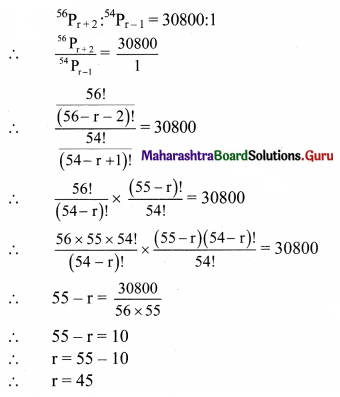Question 2.
How many words can be formed by writing letters in the word CROWN in a different order?
Solution:
Five Letters of the word CROWN are to be permuted.
∴ Number of different words = 5! = 120Question 3.
Find the number of words that can be formed by using all the letters in the word REMAIN. If these words are written in dictionary order, what will be the 40th word?
Solution:
There are 6 letters A, E, I, M, N, R.
Number of words that can be formed by using all these letters = 6! = 720
When a word starts with ‘A’,
‘A’ can be arranged in 1 way and the remaining 5 letters can be arranged among themselves in 5! ways.
The number of words starting with A = 5!
∴ Similarly,
The number of words starting with E = 5!
The number of words starting with I = 5!
The number of words starting with M = 5!
The number of words starting with N = 5!
The number of words starting with R = 5!
Total number of words = 6 × 5! = 720
Number of words starting with AE = 4! = 24
Number of words starting with AIE = 3! = 6
Number of words starting with AIM = 3! = 6
Number of words starting with AINE = 2!
Total words = 24 + 6 + 6 + 2 = 38
39th word is AINMER
40th word is AINMRE

Question 4.
The Capital English alphabet has 11 symmetric letters that appear the same when looked at in a mirror. These letters are A, H, I, M, O, T, U, V, W, X, and Y. How many symmetric three letters passwords can be formed using these letters?
Solution:
There are 11 symmetric letters.
∴ Number of 3 Letter passwords = 11P3
= 11 × 10 × 9
= 990

Question 5.
How many numbers formed using the digits 3, 2, 0, 4, 3, 2, 3 exceed one million?
Solution:
A number that exceeds one million is to be formed from the digits 3, 2, 0, 4, 3, 2, 3.
Then the numbers should be any number of 7 digits which can be formed from these digits.
Also, among the given numbers 2 is repeated twice and 3 is repeated thrice.
∴ Required number of numbers = Total number of arrangements possible among these digits – number of arrangements of 7 digits which begin with 0.
= $$\frac{7 !}{2 ! 3 !}-\frac{6 !}{2 ! 3 !}$$
= $$\frac{7 \times 6 \times 5 \times 4 \times 3 !}{2 \times 3 !}-\frac{6 \times 5 \times 4 \times 3 !}{2 \times 3 !}$$
= 7 × 6 × 5 × 2 – 6 × 5 × 2
= 6 × 5 × 2(7 – 1)
= 60 × 6
= 360Question 6.
Ten students are to be selected for a project from a class of 30 students. There are 4 students who want to be together either in the project or not in the project. Find the number of possible selections.
Solution:
Ten students are to be selected for a project from a class of 30 students.
Case I:
If 4 students join the project, then from remaining 26 students, rest of the 6 students are to be selected.
Which can be done in 26C6
= $$\frac{26 !}{6 !(26-6) !}$$
= $$\frac{26 \times 25 \times 24 \times 23 \times 22 \times 21 \times 20 !}{6 ! \times 20 !}$$
= 230230 ways.

Case II:
If 4 students does not join the project, then from remaining 26 students, all the 10 students are to be selected.
Which can be done in 26C10
= $$\frac{26 !}{10 !(26-10) !}$$
= $$\frac{26 \times 25 \times 24 \times 23 \times 22 \times 21 \times 20 \times 19 \times 18 \times 17 \times 16 !}{10 ! \times 16 !}$$
= 5311735 ways.
∴ Required number of selections = 26C6 + 26C10
= 230230 + 5311735
= 5541965

Question 7.
A student finds 7 books of his interest but can borrow only three books. He wants to borrow the Chemistry part II book only if Chemistry Part I can also be borrowed. Find the number of ways he can choose three books that he wants to borrow.
Solution:
There are 7 books of student’s interest, but he can borrow only three books.
He wants to borrow the Chemistry part II book only if Chemistry Part I can also be borrowed.
Consider the following table: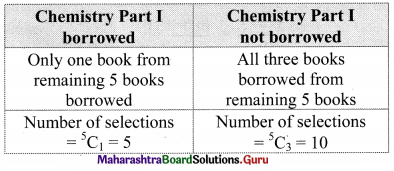Required number of selections = 5 + 10 = 15

Question 8.
30 objects are to be divided into three groups containing 7, 10, 13 objects. Find the number of distinct ways of doing so.
Solution:
First we can select 7 objects out of 30 for the first group in 30C7 ways.
Now there are 23 objects left out of which we can select 10 objects for the second group in 23C10 ways.
Remaining 13 objects can be selected for the third group in 5C5 ways.
∴ Required number of ways = 30C7 × 23C10 × 13C13
= $$\frac{30 !}{23 ! 7 !} \times \frac{23 !}{10 ! 13 !} \times 1$$
= $$\frac{30 !}{7 ! 10 ! 13 !}$$Question 9.
A student passes an examination if he secures a minimum in each of the 7 subjects. Find the number of ways a student can fail.
Solution:
Every subject a student may pass or fail.
∴ Total number of outcomes = 27 = 128
This number includes one case when the student passes in all subjects.
Required number of ways = 128 – 1 = 127

Question 10.
Nine friends decide to go for a picnic in two groups. One group decides to go by car and the other group decides to go by train. Find the number of different ways of doing so if there must be at least 3 friends in each group.
Solution:
Nine friends decide to go for a picnic in two groups and there must be at least 3 friends in each group.
Consider the following table: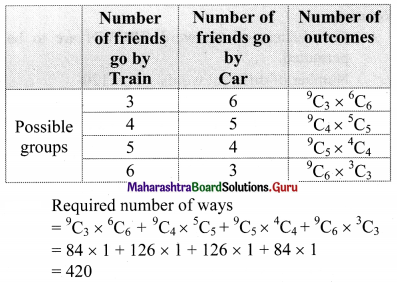Question 11.
A hall has 12 lamps and every lamp can be switched on independently. Find the number of ways of illuminating the hall.
Solution:
Every lamp is either ON or OFF.
There are 12 lamps
Number of instances = 212
This number includes one case in when all 12 lamps are OFF.
∴ Required Number of ways = 212 – 1 = 4095

Question 12.
How many quadratic equations can be formed using numbers from 0, 2, 4, 5 as coefficients if a coefficient can be repeated in an equation?
Solution:
A quadratic equation is to be formed using numbers 0, 2, 4, 5 as coefficients and a coefficient can be repeated.
Let the quadratic equation be ax2 + bx + c = 0, a ≠ 0
Consider the following table: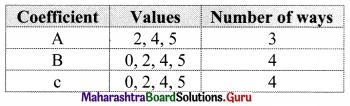Number of quadratic equations can be formed = 3 × 4 × 4 = 48

Question 13.
How many six-digit telephone numbers can be formed if the first two digits are 45 and no digit can appear more than once?
Solution:
There are total of 10 digits.
Let the telephone number be 45abcd.
There are 8 digits left for the choice of a, b, c, d as repetition is not allowed.
Consider the following table: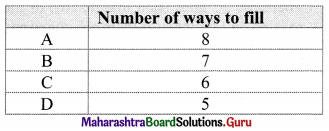∴ Required number of numbers formed = 8 × 7 × 6 × 5 = 1680Question 14.
A question paper has 6 questions. How many ways does a student have to answer if he wants to solve at least one question?
Solution:
Every question is ‘SOLVED’ or ‘NOT SOLVED’.
There are 6 questions.
Number of outcomes = 26
This number includes one case when the student solves NONE of the questions.
∴ Required number of ways = 26 – 1 = 64 – 1 = 63

Question 15.
Find the number of ways of dividing 20 objects into three groups of sizes 8, 7, and 5.
Solution:
First we can select 8 objects our of 20 for the first group in 20C8 ways.
Now there are 12 objects left out of which we can select 7 objects for the second group in 12C7 ways.
Remaining 5 objects can be selected for the third group in 5C5 ways.
∴ Required number of ways = 20C8 × 12C7 × 5C5
= $$\frac{20 !}{8 ! 12 !} \times \frac{12 !}{7 ! 5 !} \times 1$$
= $$\frac{20 !}{8 ! 7 ! 5 !}$$

Question 16.
There are 4 doctors and 8 lawyers in a panel. Find the number of ways for selecting a team of 6 if at least one doctor must be in the team.
Solution:
There are 4 doctors and 8 lawyers in a panel.
A team of 6 with at least one doctor is to be formed.
We count the number by the INDIRECT method of counting.
Number of ways to select a team of 6 people = 12C6
Number of teams with No doctor in any team = 8C6
∴ Required number of ways = 12C68C6
= 924 – 28
= 896

Question 17.
Four parallel lines intersect another set of five parallel lines. Find the number of distinct parallelograms formed.
Solution:
The first set has 4 parallel lines and another set has 5 parallel lines.
To form a parallelogram, we need 2 lines from each set.
∴ Required number of distinct parallelograms formed = 4C2 × 5C2
= 6 × 10
= 60Question 18.
There are 12 distinct points A, B, C, …, L, in order, on a circle. Lines are drawn passing through each pair of points.
(i) How many lines are there in total?
(ii) How many lines pass through D?
(iii) How many triangles are determined by lines?
(iv) How many triangles have on vertex C?
Solution:
(i) We need two points to draw a line.
∴ Total number of lines = 12C2 = 66

(ii) Lines are drawn passing through each pair of points.
∴ Lines from point D will pass through all the remaining 11 points.
∴ 11 lines pass through D.

(iii) We need three points to draw a triangle.
∴ Number of triangles = 12C3 = 220

(iv) To get the triangles with one vertex as C,
we need two vertices from the remaining 11 vertices.
∴ Number of triangles with vertex at C = 11C2
= $$\frac{11 \times 10}{2}$$
= 55